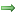# Lab Exercise for Assignment 5

## COMP102/112 2022 Tri 1: Lab Exercise 5

#### Goals

This assignment will give you more experience in writing programs that read data from a file and do something with the data. It will also give you more practice using conditionals and loops.

#### Preparation

Download the zip file for lab exercise 5 and extract it to the `COMP-102-112-2022T1-Lab5` folder in your home folder. It should contain templates for the exercises program, along with the data files. Read through the exercises and run or view the demo so that you know what you need to do.

Later when the model answer to assignment 4 becomes available, make sure you understand all the components of the programs.

Also, go over the code examples from the lectures that read files.

#### Summary

• Files data exercise:Series of programs to read data from files and print/summarise the results.
• Files draw exercise:Series of programs to read data from files and draw the results.

### Exercise Series 1: Reading Data from files

The first series of exercises is `FilesDataExercise`. It is related to `GraphPlotter` program of the assignment.

• If you are using a computer in a lab, you can run the FilesDataExercise demo to see what your program should do (look in the assig5-demos folder).
• Or, you can watch the demo video. (Note that this video includes two methods that are not part of this year version of the exercise)

The file `FilesDataExercise.java` contains three methods that each involve reading some data from a file and doing something with the data.

• `sumNumbers` should add up all the numbers in a file of numbers called numbers.txt, and print the total at the end. There may be any number of numbers on each line.
There is only one number per line.
It will need to convert the String into a double.
The total should be 2174.
• `countLargeCourses` should read data from a file called course-counts.txt that has a coursecode and a course size (the number of students) on each line. The method should count (and print out) how many of the courses have more than 80 students. (The answer should be 13.)
It will need to use a Scanner on each line in order to extract the coursecode and the course size.

• `computeTotalOrder` should read data from a file called order.txt that has an item, a count and a unit-price on each line. The method should calculate the cost of each item (count * unit-price) and print the total cost of the order. (The total should be \$167.99.)
It will need to use a Scanner on each line in order to extract the count and the unit-price.

### Exercise Series 2: Reading Data for Drawing

The second of exercises is `FilesDrawExercise`. It is closely related to the `WeatherReporter` program. All three exercises will help you with this part of the assignment.

• If you are using a computer in a lab, you can run the FilesDataExercise demo to see what your program should do (look in the assig5-demos folder).
• Or, you can watch the demo video. (Note that this video includes one method that is not part of this year version of the exercise)

The file `FilesDrawExercise.java` contains two methods that each involve reading some data from a file that can have a header, and draw pictures.

#### Hints about Colours:

You can use `UI.setColor(new Color(r, g, b))` to set the color of the UI to a new colour if `r`, `g`, and `b` are integers containing the red, green, and blue component values. `r`, `g`, and `b` must be between 0 and 255 (inclusive).

#### DotDrawer

The method `drawDots()` will read a sequence of positions and colors of dots from a file and then progressively draw the dots on the graphics pane.

Each line of the file has five numbers:
• coordinates of the center of the dot (two doubles) and
• red, green, blue (three integers)

There are three data files you can use: dots1.txt, dots2.txt, dots3.txt.

The `drawDots()` method should
• Ask user for a file name, and read all lines from the file
• For each line,
• extract the coordinates and the colour,
• draw a dot (diameter SIZE) of the specified colour centered at the specified coordinates
• sleep for 20 milliseconds

#### CirclesAndSquares

The method `drawCirclesAndSquares()` will read a sequence of positions and sizes from a file and draw blue circles and red squares at those positions.

The first line of the file contains two numbers: the number of circles and the number of squares to draw.
The remaining lines each have three numbers:
• the coordinates of the center of the shape (x, y)
• the size of the shape (diameter/width)

There are three data files you can use: C-and-S-11.txt, C-and-S-18.txt, C-and-S-33.txt. The files have the same data, but the first one specifies 11 circles and 27 squares; the second has 18 circles and 20 squares, and the third has 33 circles and 5 squares.

The `drawCirclesAndSquares()` method should:
• Ask user for a file name, and open the file with a Scanner
• First line has two numbers -
• Each remaining line has the center coordinates (x,y) and size of a shape.
• Read the header line (number of circles and number of squares)
• Read centers and sizes of each of the circles, and draw filled circles (in blue)
• Read centers and sizes of each of the squares, and draw filled squares (in red)